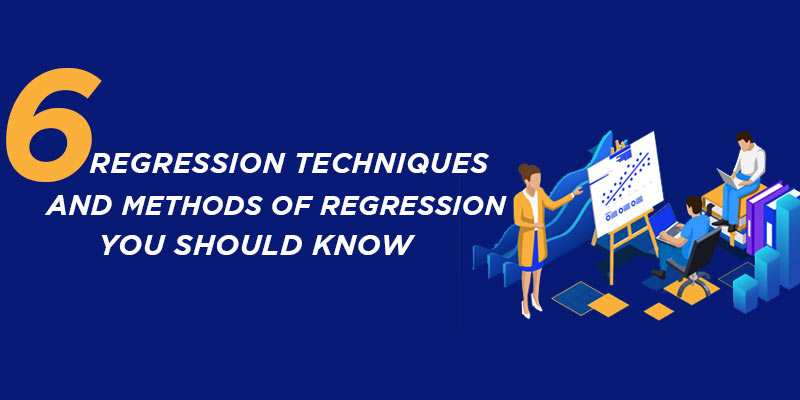Among various lucrative jobs in the IT sector, data science is an evolving and fast-growing, demanding technology. As data science helps businesses monitor, manage, and gather performance measures to improve decision-making across the organization. Most business decisions these days are data-driven. Regression Analysis is one of the most significant types of data analysis in this sector.

If you want to begin your career in data science, you can join Data Science Online Course and learn the Data Science Life Cycle, Machine Learning, regression in data science, and regression modeling in data analysis.

In this blog, we shall discuss the method of regression, regression technique, and what is a regression in data science.

The two types of regression analysis methods utilized to solve the regression problem using machine learning are logistic regression and linear regression. They are the most well-known methods of regression. However, there are other kinds of regression analysis approaches in machine learning, and their use differs depending on the data being used.

## What is Regression Analysis?

Regression analysis examines the relationship between a dataset’s target or dependent variable and the independent variable as a predictive modeling tool. Regression analysis techniques are applied when the relationship between the target and independent variables is linear or nonlinear, and the target variable has continuous values. Regression analysis is frequently used to identify cause and effect relationships, anticipate trends, time series, and predictor strength.

Regression analysis is the primary technique to handle regression issues in machine learning utilizing data modeling. It entails identifying the best fit line, which is a line that passes through all of the data points with the least possible gap between the line and each data point.

If you want to understand machine learning concepts, you can join Machine Learning Course in Chennai and learn Linear regression, estimator bias, and variance, active learning, Kernel regression, and many more.

## Types of Regression Analysis Techniques

Regression analysis approaches come in a vast range, and the number of components will determine which technique is used. Examples of these variables are:

• The kind of target variable.
• The pattern of the regression line.
• The number of independent variables

If you want to have an in-depth understanding of the method of regression, you can join Data Science Course in Chennai and learn the regression modeling in data analysis, Machine Learning Models, Data Visualization with R, and many more.

Now, we shall discuss the types of regression techniques in detail:

• Linear Regression
• Logistic Regression
• Ridge Regression
• Lasso Regression
• Polynomial Regression
• Bayesian Linear Regression

### Linear Regression

One of the most fundamental types of regression in machine learning is linear regression. A predictor and dependent variables that are linearly related to one another make up the linear regression model. Numerous linear regression models are denoted as linear regression when multiple independent variables are included in the data.

The linear regression model is represented by the equation shown below:

y=mx+c+e

The slope of the line is the m

Intercept is a c

Error in the model represents the e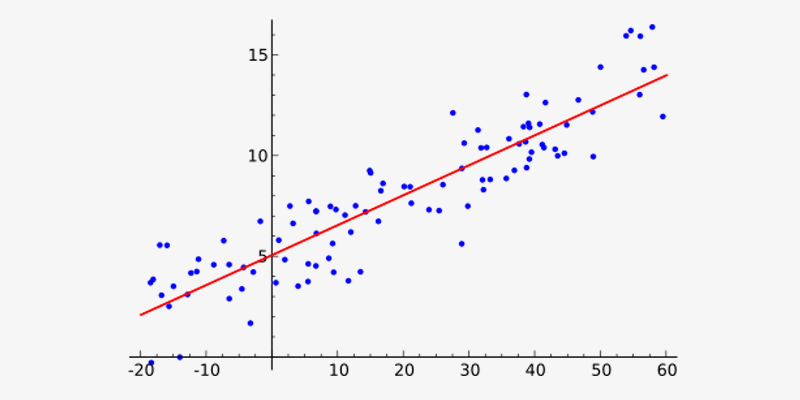The fit line is found by changing the values of m and c. The prediction error is the difference between the observed data and the predicted value. The values of m and c are chosen to obtain the least prediction error. A simple linear regression model is vulnerable to outliers; it is vital to remember this. Therefore, it shouldn’t be used when dealing with large amounts of data.

### Logistic Regression

When the dependent variable is discrete, one of the regression analysis methods employed is logistic regression. The target variable can only take on two values, and a sigmoid curve represents the relationship between the target and independent variables.

In logistic regression, the logit function is used to quantify the connection between the dependent and independent variables. The logistic regression equation is shown below.

logit(p) = ln(p/(1-p)) = b0+b1X1+b2X2+b3X3….+bkXk

where p is the probability of occurrence of the feature.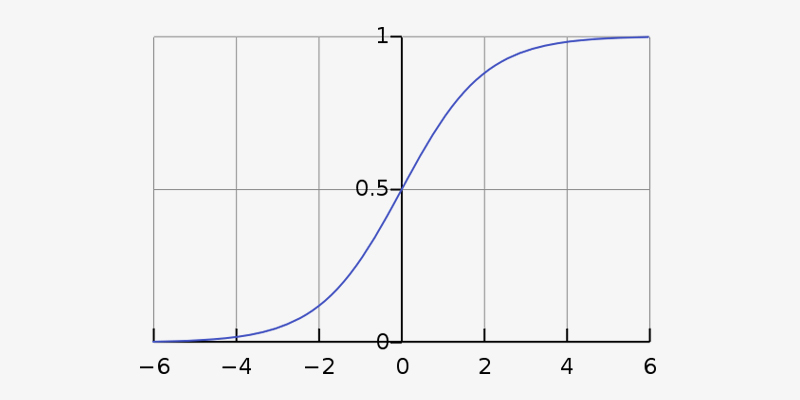To have a comprehensive understanding of regression in data science, you can join

Data Science Courses In Bangalore and impart yourself with the advanced regression techniques, Pie Charts and Bar Charts, Box Plots and Scatter Plots, and Histograms and Line Graphs.

### Ridge Regression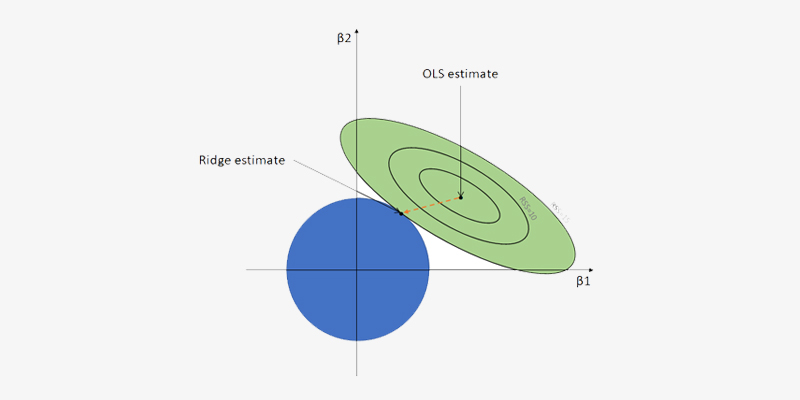Another regression type utilized in machine learning is typically applied when the correlation between the independent variables is significant.

The least-square estimates provide unbiased results for multi-collinear data. However, there may be a bias value if the collinearity is relatively high. As a result, the Ridge Regression equation includes a bias matrix. With this effective regression technique, the model is less prone to overfitting.

The Ridge Regression equation is shown below. By adding (lambda), the multicollinearity issue is resolved.

β = (X^{T}X + λ*I)^{-1}X^{T}y

### Lasso Regression

Lasso Regression is one of the regression models used in machine learning that combines feature selection and regularisation. As a result, unlike in the case of Ridge Regression, the coefficient value reaches zero.

As a result, Lasso Regression uses feature selection, which enables choosing a set of features from the dataset to build the model. Only the necessary characteristics are used in Lasso Regression, and the rest are set to zero. This aids in preventing the model from becoming overfitting. Lasso regression selects just one variable when the independent variables are strongly collinear, and it causes the other variables to be reduced to zero.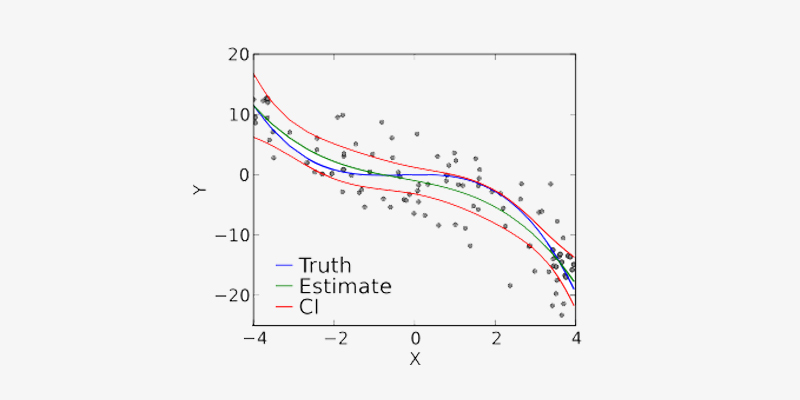The equation for the Lasso Regression technique is shown below:

N^{-1}Σ^{N}_{i=1}f(x_{i}, y_{I}, α, β)

Join Machine Learning Course in Bangalore and learn Linear Classification, Learning Bayesian networks, Classification errors, and Logistic regression.

Polynomial Regression

Another regression analysis technique in machine learning is polynomial regression, the same as multiple linear regression with a few minor changes. The n-th degree in polynomial regression defines the link between the independent and dependent variables, X and Y.

As an estimator, it uses a linear model. Polynomial regression also uses the Least Mean Squares method. The curve of the best-fit line in polynomial regression, which depends on the power of X or the number of n, crosses all data points instead of straight lines.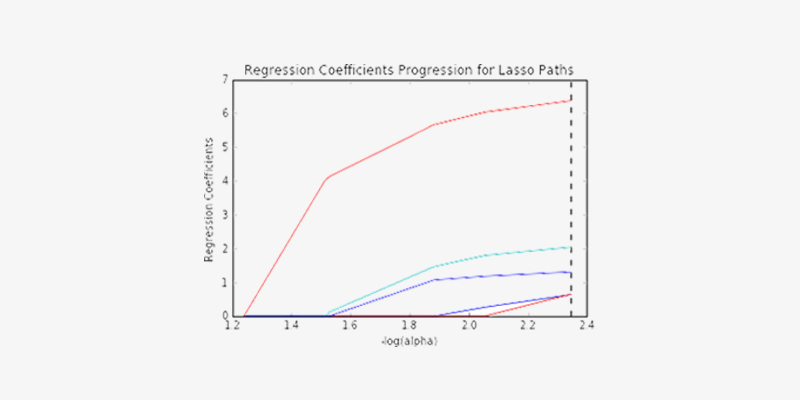The model may be prone to overfitting while attempting to obtain the greatest fit line and a Minimum Mean Squared Error. The higher polynomials can extrapolate to unusual results. Thus it is recommended to observe the curve at the end.

The below equation represents the Polynomial Regression:

l = β0+ β0x1+ε

### Bayesian Linear Regression

One of the regression models used in machine learning, Bayesian Regression, calculates the value of the regression coefficients using the Bayes theorem. Instead of locating the least squares, this regression method determines the posterior distribution of the features. Similar to both linear and ridge regression, bayesian linear regression is more stable than simple linear regression.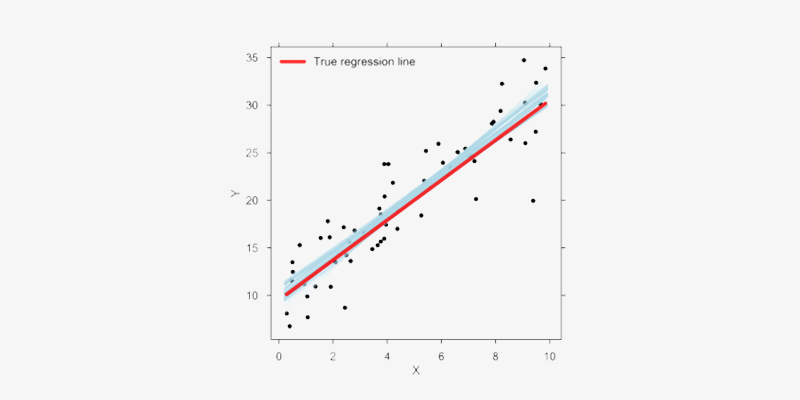Now, you would have understood the method of regression, regression technique, and regression in data analytics. So, to have a comprehensive understanding of advanced regression techniques, you can join a Data Science Course in Coimbatore and learn regression techniques, Hypothesis Testing, Various Machine Learning Models, and many more concepts.